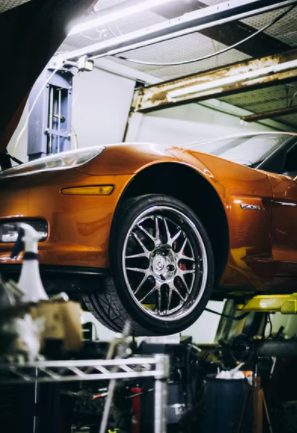## Car Lifts

Consider an auto-repair shop has a hydraulic lift, with a cylindrical diameter of 0.2 m, and weighs 40 kg (piston + arms set up). If a customer has a 700 kg car, what pressure should the mechanic pump the hydraulic fluid to suspend the vehicle? Assume normal atmospheric pressure of 101 kPa.Hint
$$Force=mass \times acceleration$$$Hint 2 $$Pressure=\frac{Force}{Area}+P_{atm}$$$
where $$P_{atm}$$ is the atmospheric pressure.
Gravity is the force acting on the mass and system. Assuming the y-direction is on the axis of the piston:
$$\sum F_y=ma\rightarrow F=(m_{arms}+m_{car})(9.81\frac{m}{s^2})$$$where $$F$$ is the force, $$m$$ is the mass, and $$a$$ is the acceleration due to gravity. $$F=(740kg)(9.81\frac{m}{s^2})=7259.4\:N$$$
Now, balance this force with the pressure force:
$$F=(P-P_{atm})(Area)=7259.4N$$$Rearranging the equation to solve for pressure: $$P=\frac{F}{A}+P_{atm}$$$
where area is:
$$A=\frac{\pi d^2}{4}=\frac{\pi (0.2m)^2}{4}=0.0314m^2$$$Thus, $$P=\frac{F}{A}+P_{atm}=\frac{7259.4N}{0.0314m^2}+101\:kPa=332\:kPa$$$
332 kPa